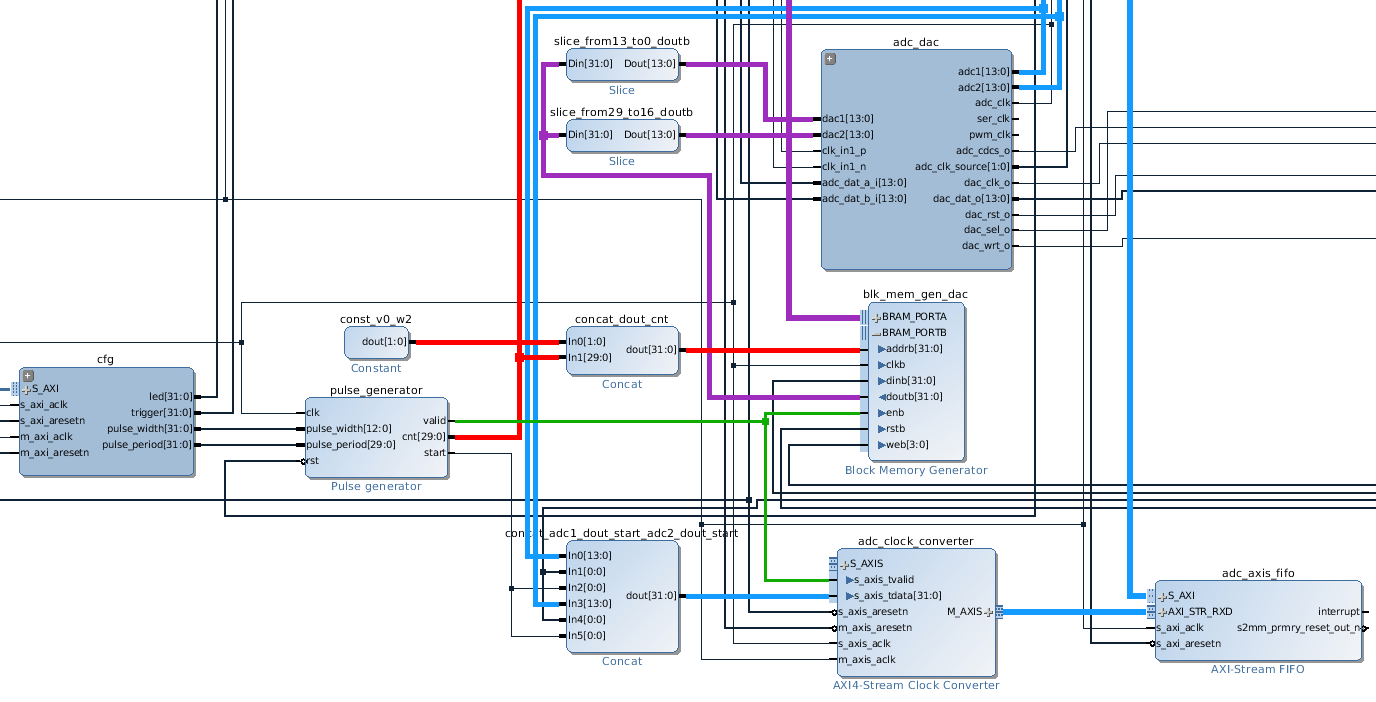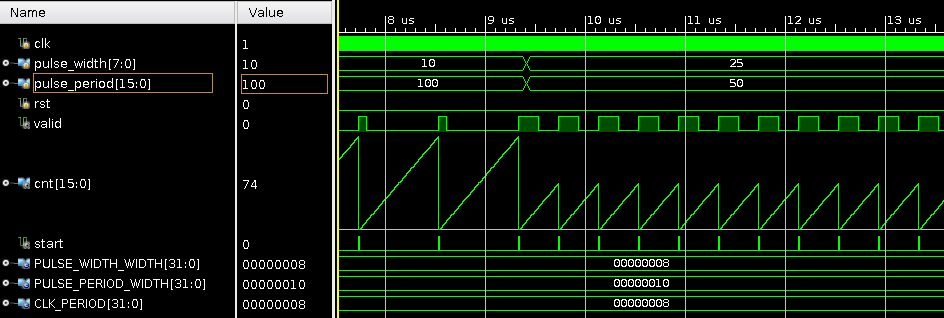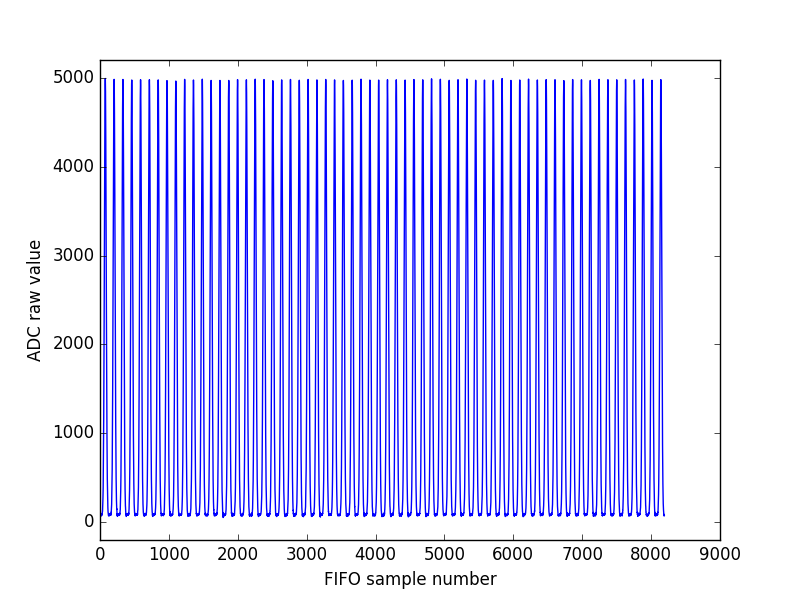Our office will be closed from December 20, 2023 to January 02, 2024. Orders placed during this time will be processed starting on January 03, 2024.

# Pulse generator for the Red Pitaya

2016-10-13

We present the design of a simple pulse generator for the Red Pitaya. The design uses standard Xilinx IP blocks and a custom Verilog core that outputs a signal valid that is driven high when the pulse is played on the DAC.

Pulse shape is stored in a Block RAM that can be written from Linux. ADC data is written in a FIFO that can be read from Linux. The FIFO only accepts ADC data when a pulse is played on the DAC.

The valid signal is shown in green in the figure below. It is connected to the DAC Block RAM and the ADC FIFO.

The ouput port doutb of the DAC BRAM is 32 bits wide. doutb[13:0] and doutb[29:16] are respectively connected to DAC1 and DAC2 (purple). Note that the pulse_generator core also outputs a signal cnt that increments at each clock cycle when valid is high. The signal is used to specify the address of the data that should be played by the BRAM (red).The blue connections show the ADC data path. The FIFO has a width of 32 bits, which allows to store the two ADC channels plus a start signal that identifies the beginning of a pulse. An AXI clock converter is used to move data from the ADC clock domain (125 MHz) to the interconnect clock domain (200 MHz).

## Verilog core

We wrote a simple Verilog core to generate a digital pulse of specified width and period, as shown in the simulation below:The core consists of a counter cnt_reg that increments between 0 and pulse_period - 1 at each clock cycle. The value of cnt_reg is compared to pulse_width to obtain the valid signal:

timescale 1 ns / 1 ps

module pulse_generator #
(
parameter integer PULSE_WIDTH_WIDTH = 8,
parameter integer PULSE_PERIOD_WIDTH = 16
)
(
input wire clk,
input wire [PULSE_WIDTH_WIDTH-1:0] pulse_width,
input wire [PULSE_PERIOD_WIDTH-1:0] pulse_period,
input wire rst,
output reg valid,
output reg [PULSE_PERIOD_WIDTH-1:0] cnt,
output reg start
);

reg rst_reg;
reg [PULSE_PERIOD_WIDTH-1:0] cnt_reg;
initial cnt_reg = 0;

always @(posedge clk) begin
start <= (cnt_reg == 0);
valid <= (cnt_reg < pulse_width);
rst_reg <= rst;
cnt <= cnt_reg;
end

always @(posedge clk) begin
if (!rst_reg & rst) begin
cnt_reg <= 0;
end else begin
if (cnt_reg < pulse_period - 1) begin
cnt_reg <= cnt_reg + 1;
end else begin
cnt_reg <= 0;
end
end
end

endmodule
`

## Controlling the instrument with Python

Below is a screenshot of a simple Python Matplotlib animation that displays the acquired pulses. Pulse width is 128 (1.024 µs) and pulse period is 125 000 (1 ms i.e. pulse rate of 1 kHz). The animation runs displays the last 64 contiguous pulses gathered from the FIFO every 64 ms.## FPGA design source code

https://github.com/Koheron/koheron-sdk/tree/master/examples/red-pitaya/pulse-generator